Courses

# Thermodynamic Relations - 1

## 10 Questions MCQ Test Topicwise Question Bank for GATE Mechanical Engineering | Thermodynamic Relations - 1

Description
This mock test of Thermodynamic Relations - 1 for Mechanical Engineering helps you for every Mechanical Engineering entrance exam. This contains 10 Multiple Choice Questions for Mechanical Engineering Thermodynamic Relations - 1 (mcq) to study with solutions a complete question bank. The solved questions answers in this Thermodynamic Relations - 1 quiz give you a good mix of easy questions and tough questions. Mechanical Engineering students definitely take this Thermodynamic Relations - 1 exercise for a better result in the exam. You can find other Thermodynamic Relations - 1 extra questions, long questions & short questions for Mechanical Engineering on EduRev as well by searching above.
QUESTION: 1

Solution:
QUESTION: 2

Solution:
QUESTION: 3

### A gas having a negative Joule-Thomson coefficient ( μj < 0), when throttled, will

Solution: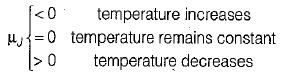QUESTION: 4

The Clausius-Clapeyron equation gives the slope of curve on

Solution: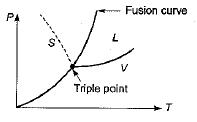QUESTION: 5

The variation of saturation pressure with saturation temperature for a liquid is 0.1 bar/K at 400 K. The specific volume of saturated liquid and dry saturated vapour at 400 K are 0.251 and 0.001 m3/kg. What will be value of latent heat of vaporization using Clausius clapeyron equation?

Solution: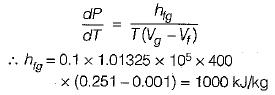QUESTION: 6

Consider the following statements pertaining to the Clapeyron equation:
1. It is useful in estimating properties like enthalpy from other measurable properties.
2. At a change of phase, it can be used to find the latent heat at a given pressure.
3. It is derived from the relationship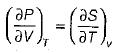Which of the statements given above are correct?

Solution:
QUESTION: 7

Which of the following equation is Clapeyron- Clausius equation?

Solution:
QUESTION: 8

At chemical equilibrium, Gibbs function is

Solution:

(dG)T,P = 0 (equilibrium condition)

QUESTION: 9

Which of the following parameter is.the criterion of equilibrium and stability of a system existing at constant volume and constant temperature?

Solution: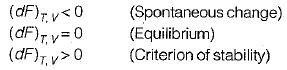QUESTION: 10

The internal energy of an ideal gas is a function of

Solution:

For an ideal Gas
U = f(T) only